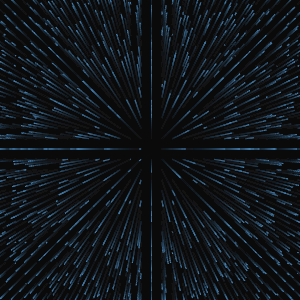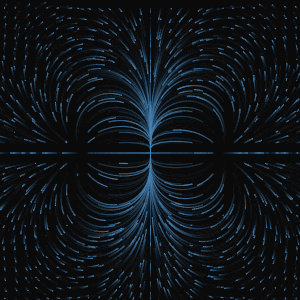### Free Ginckers

#### From Vector Potential: Two Current Wires

Template: Graphics/vector-chart-from-vector-potential

Discription: Vector chart of the magnetic field distribution created using the vector potential for a pair of long parallel current wires.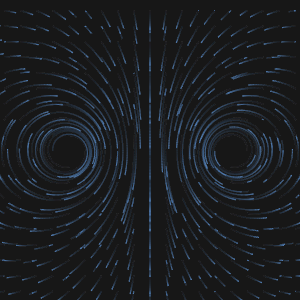#### From Vector Potential: Current Wire

Template: Graphics/vector-chart-from-vector-potential

Discription: Vector chart of the magnetic field distribution created using the vector potential for a current wire.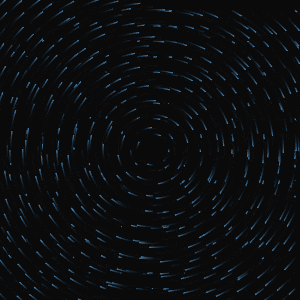#### Vector Chart from Field: A Wire Current

Template: Graphics/vector-chart-from-field

Discription: Vector chart created using the field components for a wire current (magnetic field distribution).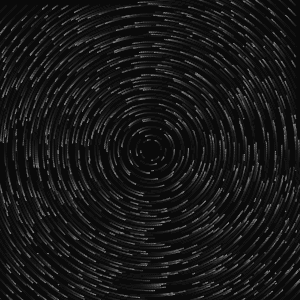#### Vector Chart from Field: Cosine, Sine

Template: Graphics/vector-chart-from-field

Discription: Vector chart created using the field components for a cosine-sine field.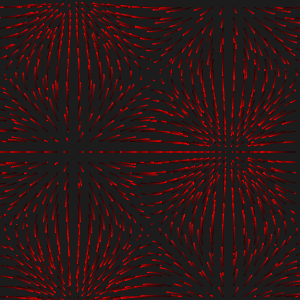#### Vector Chart from Field: Electric Dipole

Template: Graphics/vector-chart-from-field

Discription: Vector chart created using the field components for an electric dipole field.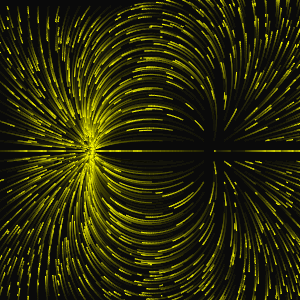#### Vector Chart from Field: Point Charge

Template: Graphics/vector-chart-from-field

Discription: Vector chart created using the field components for a point charge.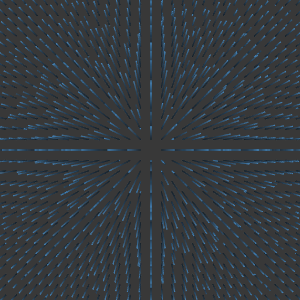#### Vector Chart from Field: Wind Model

Template: Graphics/vector-chart-from-field

Discription: Vector chart created using the field components for a wind model.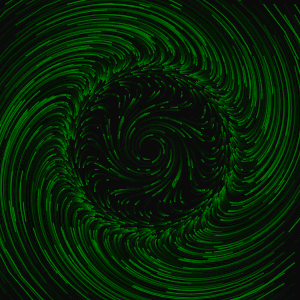#### Vector Chart from Field: X-Y Field

Template: Graphics/vector-chart-from-field

Discription: Vector chart created using the field components for an X-Y field.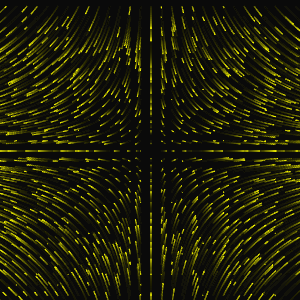#### Vector Chart from Potential: a*y*sin(x) + b*x*y^2

Template: Graphics/vector-chart-from-potential

Discription: Vector chart created using potential for a potential function of a*y*sin(x) + b*x*y^2.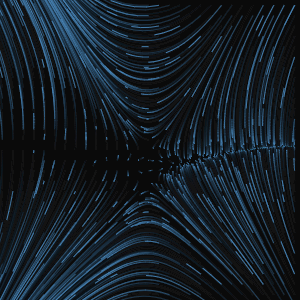#### Vector Chart from Potential: Charge Rod

Template: Graphics/vector-chart-from-potential

Discription: Vector chart created from potential for a charge rod.#### Vector Chart from Potential: Electric Dipole

Template: Graphics/vector-chart-from-potential

Discription: Vector chart created using potential for an electric dipole.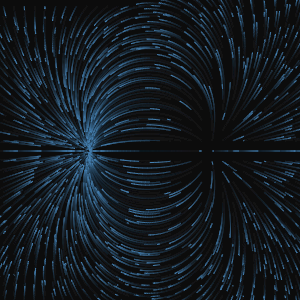#### Vector Chart from Potential: Octupole

Template: Graphics/vector-chart-from-potential

Discription: Vector chart created using the potential for an electric octupole.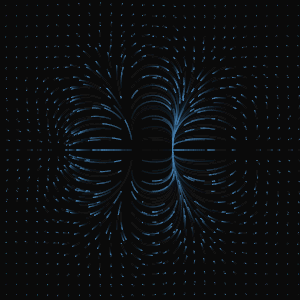#### Vector Chart from Potential: Point Charge

Template: Graphics/vector-chart-from-potential

Discription: Vector chart created using potential for a point charge.